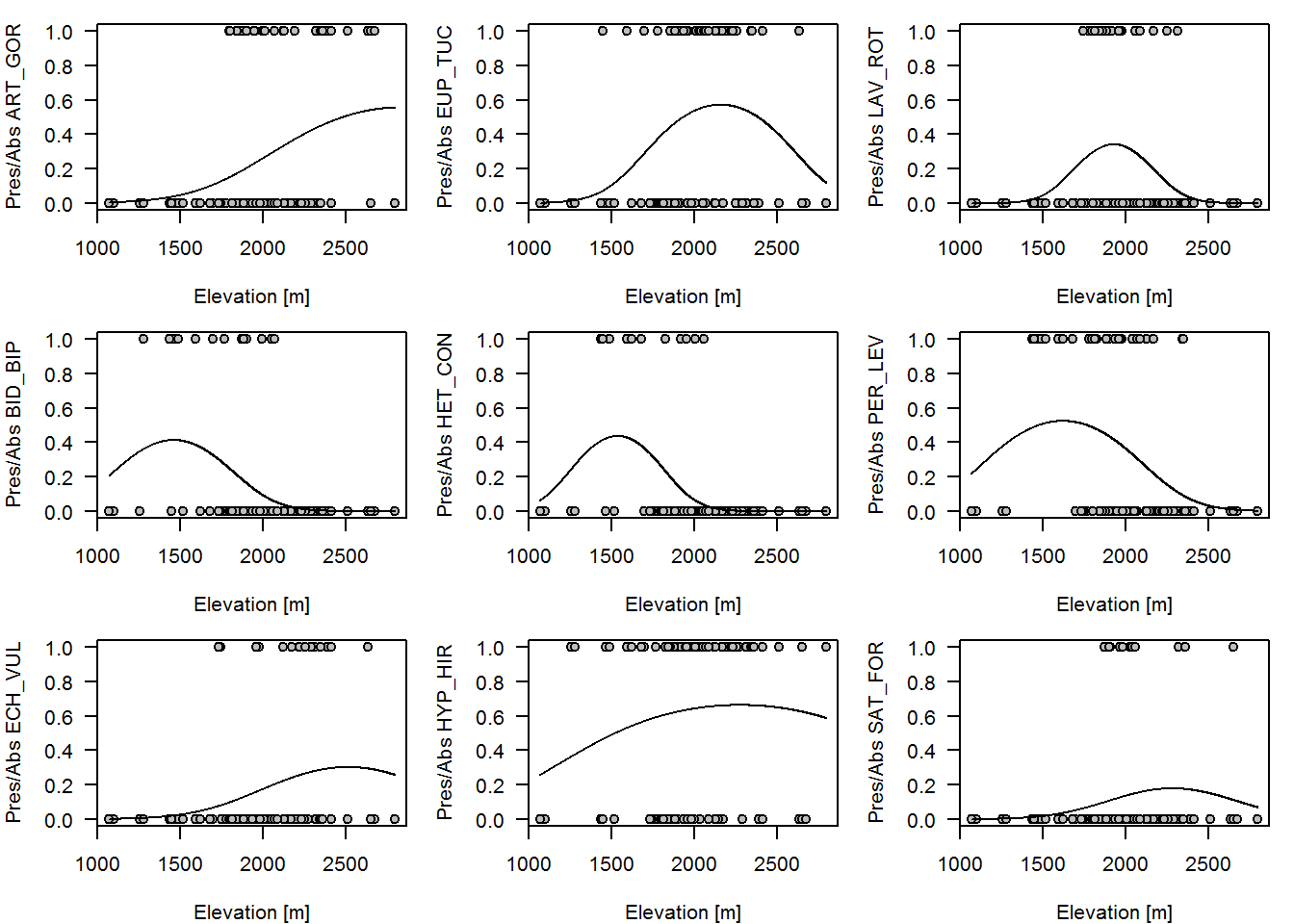#BIS-Fogo

### Site Tools

en:learning:schools:s02:code-examples:sm-ce-03-02

## C01-2: Binominal analysis

The following example uses data from a field survey of areas in the Fogo natural park in 2007 by K. Mauer. For more information, please refer to this report.

#### Occurrence of species as a function of elevation

Again, we assume that elevation has some explanatory power. This time, the elevational distribution of selected species should be analyzed.

To visualize the results, a binary plot (i.e. presence/absence) is computed for each species and a binominal generalized linear model is fitted to this distribution using elevation (and it’s square) as explanatory variable.

In order to compute this for 8 species (defined in variable “species_considered”), a for-loop is used. Within each loop, a plot for the respective species is generated and a GLM model is fitted afterwards. To get the elevational function of species occurrence, the fitted model is used to predict the respective occurrence at height intervals of 1 meter between the minimum and maximum elevation for which species data has been recorded.

# Set graphical output to 3 by 3 columns/rows
par(mar=c(4,4,1,1),las=1,cex=0.9,mfcol=c(3,3))

# Store elevation in variable x (just for technical reasons of the prediction
# function)
x <- data$ALT_GPS_M predict_x <- min(x):max(x) # Compute elevational distribution for each species stored in variable # species_considered for (i in species_considered) { # Compute plot plot(data$ALT_GPS_M, data_species[,i]>0,
xlab="Elevation [m]",ylab=paste("Pres/Abs",names(data_species)[i]),
pch=21,bg="grey")

# Train model
model <- glm((data_species[,i]>0) ~ x + I(x^2),family="binomial")

# Use model to predict species elevational distribution
pred <- predict(model, newdata = data.frame(x = predict_x))

# Add prediction results to plots
lines(predict_x, exp(pred) / (1 + exp(pred)))
}par(user)